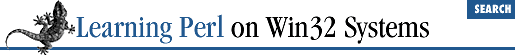home | O'Reilly's CD bookshelfs | FreeBSD | Linux | Cisco | Cisco Exam

### A.6 Chapter 7, Regular Expressions

1. Here are some possible answers:

1. ``` /a+b*/```

2. ``` /\\*\**/``` (Remember that the backslash cancels the meaning of the special character following.)

3. ``` /(\$whatever){3}/``` (You must have the parentheses, or else the multiplier applies only to the last character of ``` \$whatever``` ; this solution also fails if ``` \$whatever``` has special characters.)

4. ``` /[\000-\377]{5}/``` or ``` /(.|\n){5}/``` (You can't use dot alone here, because dot doesn't match newline.)

5. ``` /(^|\s)(\S+)(\s+\2)+(\s|\$)/``` (``` \S``` is non-whitespace, and ``` \2``` is a reference to whatever the "word" is; the caret or whitespace alternative ensures that the ``` \S+``` begins at a whitespace boundary.)

1. One way to do this is:

```     while (<STDIN>) {
if (/a/i && /e/i && /i/i && /o/i && /u/i) {
print;
}
}```

Here, we have an expression consisting of five match operators. These operators are all looking at the contents of the ``` \$_``` variable, which is where the control expression of the ``` while``` loop is putting each line. The match operator expression will be true only when all five vowels are found.

Note that as soon as any of the five vowels are not found, the remainder of the expression is skipped, because the ``` &&``` operator doesn't evaluate its right argument if the left argument is false. For more information on using the ``` &&``` operator as a control structure, please refer to the section, "&&, ||, and ?: as Control Structures" .

2. Another way to do this is:

```     while (<STDIN>) {
if (/a.*e.*i.*o.*u/i) {
print;
}
}```

This answer turns out to be easier than the other part of this exercise. Here we have a simple regular expression, that looks for the five vowels in sequence, separated by any number of characters.

3. A third way to do this is:

```     while (<STDIN>) {
if (/^[eiou]*a[^iou]*e[^aou]*i[^aeu]*o[^aei]*u[^aeio]*\$/i) {
print;
}
}```

This solution is ugly, but it works. To construct this solution, just think "What can go between the beginning of the line, and the first a?" Then, think "What can go between the first a and the first e?" Eventually, everything works out, with a little assistance from you.A.5 Chapter 6, Basic I/OA.7 Chapter 8, Functions